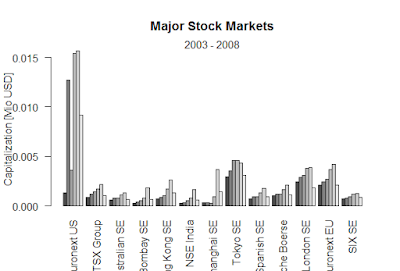Showing posts with label White Neural Network Test. Show all posts
Showing posts with label White Neural Network Test. Show all posts

## How to test nonlinearity data with white neural network.

> n <- 999
> # Get Non-linear in ``mean'' regression
> x <- runif(1000, -1, 1)
> y <- x^2 - x^3 + 0.1*rnorm(x)
> white.test(x, y)

White Neural Network Test

data:  x and y
X-squared = 2164, df = 2, p-value < 2.2e-16

> white.test(cbind(x,x^2,x^3), y)

White Neural Network Test

data:  cbind(x, x^2, x^3) and y
X-squared = 2.1795, df = 2, p-value = 0.3363

> ## Generate time series which is nonlinear in ``mean''
> x <- 0.0
> for(i in (2:n)) {
+     x[i] <- 0.4*x[i-1] + tanh(x[i-1]) + rnorm(1, sd=0.5)
+ }
> x <- as.ts(x)
> plot(x)

> white.test(x)

White Neural Network Test

data:  x
X-squared = 132.99, df = 2, p-value < 2.2e-16

### Black-Scholes formula-R

Black-Scholes formula-R > BlackScholes <- function(TypeFlag = c("c", "p"), S, X, Time, r, b, sigma) { TypeFla...